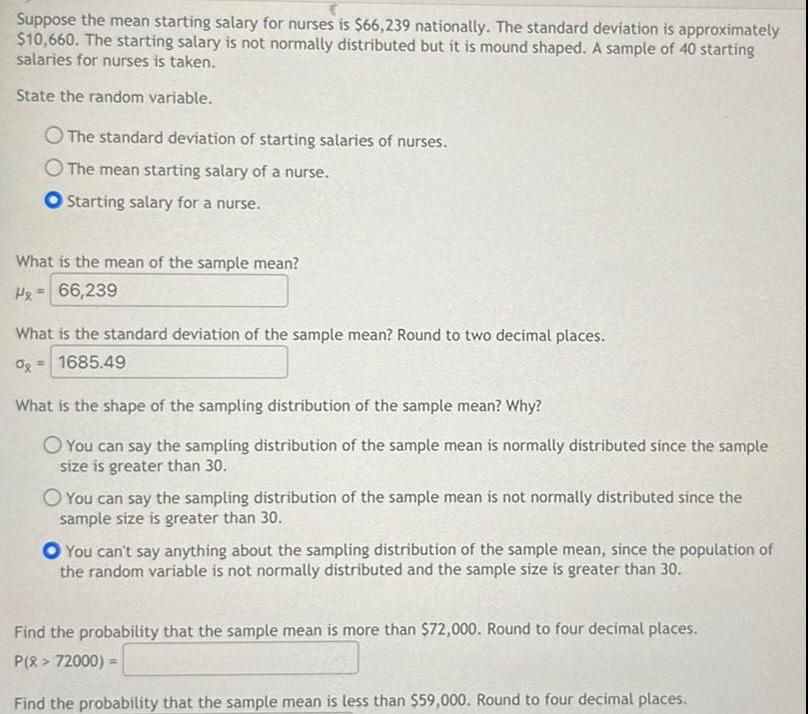Question:

# Suppose the mean starting salary for nurses is 66 239

Last updated: 11/20/2023Suppose the mean starting salary for nurses is 66 239 nationally The standard deviation is approximately 10 660 The starting salary is not normally distributed but it is mound shaped A sample of 40 starting salaries for nurses is taken State the random variable O The standard deviation of starting salaries of nurses O The mean starting salary of a nurse Starting salary for a nurse What is the mean of the sample mean H 66 239 What is the standard deviation of the sample mean Round to two decimal places 0 1685 49 What is the shape of the sampling distribution of the sample mean Why O You can say the sampling distribution of the sample mean is normally distributed since the sample size is greater than 30 O You can say the sampling distribution of the sample mean is not normally distributed since the sample size is greater than 30 O You can t say anything about the sampling distribution of the sample mean since the population of the random variable is not normally distributed and the sample size is greater than 30 Find the probability that the sample mean is more than 72 000 Round to four decimal places P X 72000 Find the probability that the sample mean is less than 59 000 Round to four decimal places